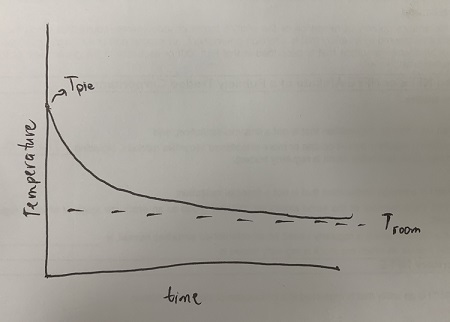# You place a frozen pie in an oven and bake it for an hour. Then you take it out and let it cool...

## Question:

You place a frozen pie in an oven and bake it for an hour. Then you take it out and let it cool before eating it. Describe how the temperature of the pie changes as time passes. Then sketch a rough graph of the temperature of the pie as a function of time.

## Newton's Law of Cooling

Newton's Law of Cooling suggests that the rate of change in temperature of a body is proportional to the difference between the temperature of the body {eq}T_b {/eq} and its surroundings {eq}T_s {/eq}. The resulting equation is of the form:

$$T_b = T_s + (T_b - T_s) e^{-kt}$$

where {eq}k {/eq} is a constant.

Since the pie is presumably warmer than room temperature, it will necessarily cool down in time. By Newton's Law of Cooling, the rate of change in the temperature of the pie is proportional to the temperature difference between the pie and the room. Thus, for the first few moments, the change in the pie's temperature will be significant, but at some point (especially when the temperature of the pie is very very close to that of room temperature), the change in temperature will be negligible.

A rough sketch of the temperature of the pie as a function of time is shown below. This is based on the resulting equation from Newton's Law of Cooling:

$$T_{\text{pie}} = T_{\text{room}}+ (T_{\text{pie}}-T_{\text{room}})e^{-kt}$$